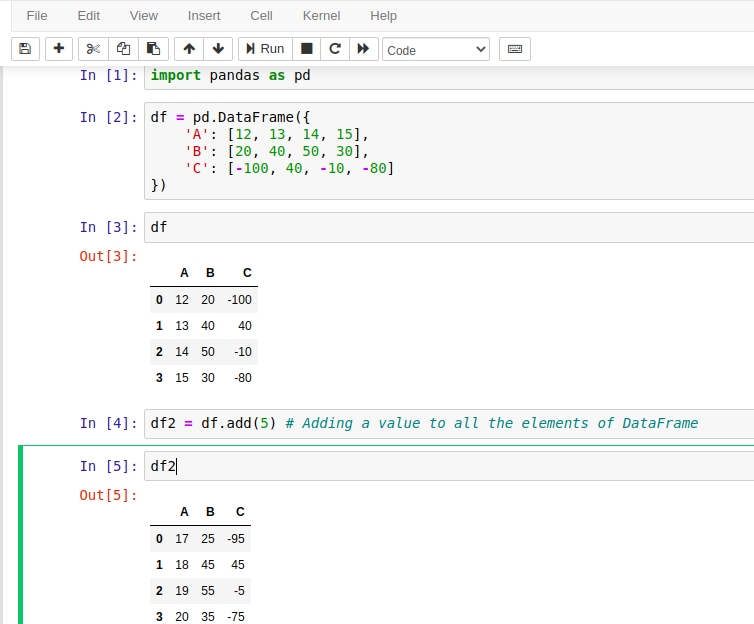Pandas add() method is a built-in method of DataFrame that is used to add data to an existing DataFrame and returns a new DataFrame. The data can be a numerical value, series or a DataFrame.

We can use the Pandas add() method to get the addition of DataFrames. It acts as binary plus(+) operator that performs arithmetic addition.

Since it performs arithmetic operations then the DataFrame must contain numerical values.

## Syntax

``DataFrame.add(other, axis='columns', level=None, fill_value=None)``

### Parameters:

other: It can be a scalar value, sequenceSeries, or a DataFrame.

axis: {0 or ‘index’, 1 or ‘columns’}. For Series input, axis to match Series index on.

level: int or label.

fill_value: Default is None but can be a float.

### Return Value:

It returns a DataFrame after performing addition operation.

## Example with Jupyter Notebook

Let's take an example to understand the use of pandas.DataFrame.add() method. Following is the code that executed by using Jupyter notebook. If you are familiar with Jupyter, See the screenshot below.## Example: How to Add Data to a DataFrame in Pandas?

We can use DataFrame.add() method to add a scalar numerical value to each element of DatafFrame. We get a DataFrame after using add() method with modified values. See the example here. This example is similar to the above code, executed by using Jupyter notebook. If you are not familiar with Jupyter then see this code to understand.

``````# import pandas library
import pandas as pd
# Create DataFrame of data
df = pd.DataFrame({
'A': [12, 13, 14, 15],
'B': [20, 40, 50, 30],
'C': [-100, 40, -10, -80]
})
# print dataframem
print(df)
print("New Dataframe after adding a value...")
# print new dataframe
print(df2)
``````

Output:

A   B    C
0  12  20 -100
1  13  40   40
2  14  50  -10
3  15  30  -80
New Dataframe after adding a value...
A   B   C
0  17  25 -95
1  18  45  45
2  19  55  -5
3  20  35 -75

## Example: Add Two DataFrames in Pandas

Apart from a scalar value, we can use DataFrame.add() method to add two DataFrames and get a new DataFrame that contains the sum of both DataFrame values. See the example here.

``````# import pandas library
import pandas as pd
# Create DataFrame of data
df = pd.DataFrame({
'A': [12, 13, 14, 15],
'B': [20, 40, 50, 30],
'C': [-100, 40, -10, 25]
})
# Create another dataframe
df2 = pd.DataFrame({
'A': [22, 23, 24, 25],
'B': [80, 40, 30, 40],
'C': [-200, 70, -50, 25]
})

print("New Dataframe after adding another dataframe...")
# print new dataframe
print(df3)
``````

Output:

New Dataframe after adding another dataframe...
A    B    C
0  34  100 -300
1  36   80  110
2  38   80  -60
3  40   70   50

## Example: Create DataFrame using Numpy in Pandas

We can use the NumPy library to create dynamic DataFrame. Here, we are using the random() method of NumPy that returns random numbers and after that by using DataFrame.add() method, we are adding 10 to each element of this DataFrame.

``````# import pandas and numpy library
import pandas as pd
import numpy as np
# Creating DataFrame using numpy Array
df = pd.DataFrame(np.random.rand(5, 3), columns =['A', 'B', 'C'])
# Print DataFrame
print(df)
# Add a value to dataframe
print("New Dataframe after adding a value ...")
# Print new dataframe
print(df2)
``````

Output:

A         B                   C
0  0.030237  0.033794  0.445364
1  0.816918  0.321307  0.059772
2  0.758902  0.472600  0.406236
3  0.512413  0.877692  0.825946
4  0.452699  0.837390  0.111349
New Dataframe after adding a value ...
A          B                    C
0  10.030237  10.033794  10.445364
1  10.816918  10.321307  10.059772
2  10.758902  10.472600  10.406236
3  10.512413  10.877692  10.825946
4  10.452699  10.837390  10.111349

## Example: Array Must have the same length of values

While working with DataFrame, make sure it contains the same length of array. Otherwise, Python raises ValueError('arrays must all be same length'). This is a common mistake we all do while working with DataFrame. See the example here.

``````# import pandas library
import pandas as pd
# Create DataFrame of data
df = pd.DataFrame({
'A': [12, 13, 14, 15],
'B': [20, 40, 50, 30],
'C': [-100, 40, -10] # less values
})
# print dataframem
print(df)
print("New Dataframe after adding a value...")
# print new dataframe
print(df2)
``````

Output:

raise ValueError('arrays must all be same length')
ValueError: arrays must all be same length

Trending